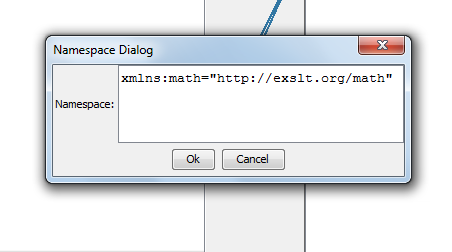# Using max() function with "XALAN" Transformer

Requirement :

You can achieve the requirement of using max() function in the data mapping having XSL transformer: "XALAN".

Solution :

Following are the high-level steps that need to follow to use max() function in Data mapping:
1. Go to the Add Namespace option, under Namespace in Actions Menu in Data Mapper applet.

2. Now, simply copy the below-mentioned namespace in the text input area and click Ok button to add the namespace to XSL permanently.
' xmlns:math="http://exslt.org/math" '3. After adding the namespace, you can use the Max function by writing the below statement in the textual rule ( or wherever you want to use ).
' math:max(XPath of element) '

For example: If you want to find out the maximum age from records then simply write it as, math:max(/Root/Record/Age).

Note: The Max function is used for a node having the data type of number or string. The maximum is the result of converting the string value of the first node in this sorted list to a number using the number function
If the node-set is empty, or if the result of converting the string values of any of the nodes to a number is NaN, then NaN is returned.